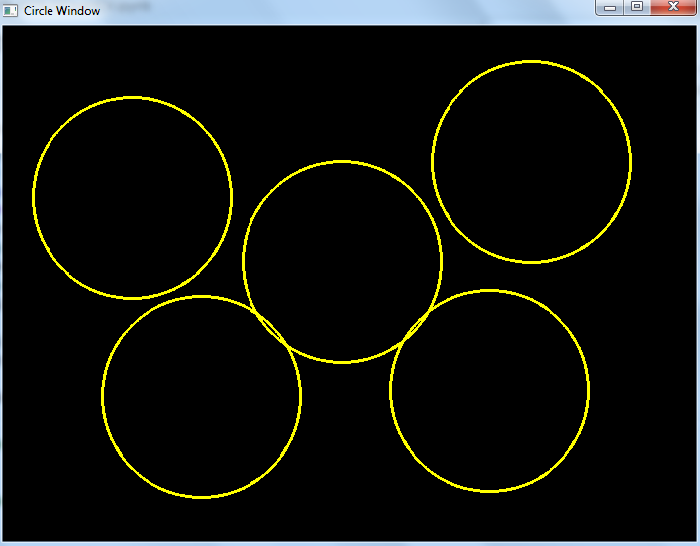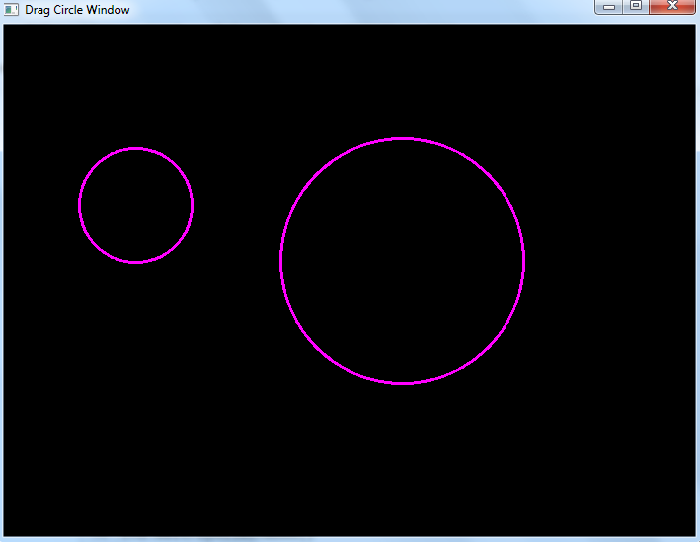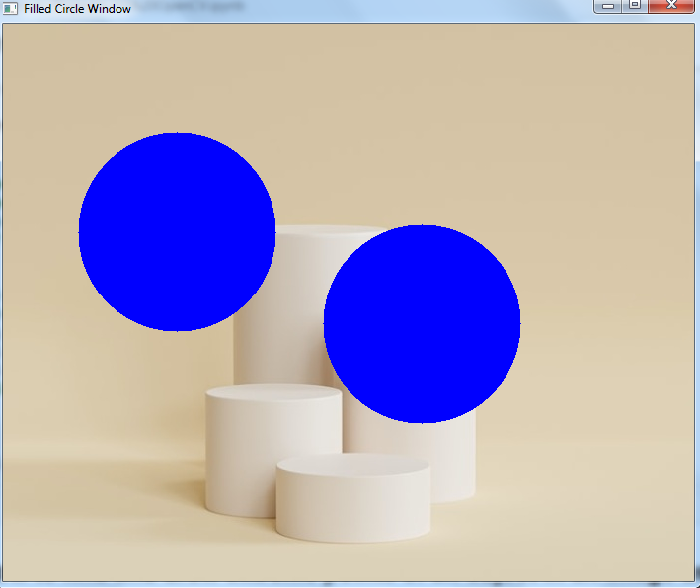# OpenCV Python ŌĆō How to draw circles using Mouse Events?

There are different types of muse events such as left or right button click, mouse move, left button double click etc. OpenCV provides us with different types of mouse events such as cv2.EVENT_LBUTTONDOWN for mouse left button down, cv2.EVENT_RBUTTONDOWN for right button down, cv2.EVENT_LBUTTONDBLCLK for left button double click and others.

A mouse event returns the coordinates (x,y) of the mouse event. To perform an action when an event happens, we define a mouse callback function. We use mouse events to draw circles on the image.

## Steps

To draw circles using mouse events, follow the steps given below ŌłÆ

• Import the required library OpenCV. Make sure you have already installed it.

• Create a black image. We draw the circles on this black image. We also read an image using cv2.imread() method to draw the circles on it.

• Define a mouse callback function to draw a circle on the image. The mouse callback function is executed when a mouse event happens. A mouse event gives the coordinate of the mouse event. Here we define a mouse callback function to draw a circle when the mouse left button is down.

• Create a window and bind the mouse callback function to this window.

• Display the image window. This window opens the image on which we draw the circle. To close the window press esc button.

Let's look at some program examples to understand how it works.

## Example

In this Python program, we draw circles when the left button of the mouse is pressed.

# Import required libraries
import cv2
import numpy as np

# define an image (black) on which the circle to be drawn
img = np.zeros((512,700,3), np.uint8)

# define mouse callback function to draw circle
def draw_circle(event, x, y, flags, param):
if event == cv2.EVENT_LBUTTONDOWN:
cv2.circle(img, (x, y), 100, (0, 255, 255), 2)

# Create a window
cv2.namedWindow("Circle Window")

# bind the callback function to the window
cv2.setMouseCallback("Circle Window", draw_circle)

# display the image
while True:
cv2.imshow("Circle Window", img)
if cv2.waitKey(20) & 0xFF == 27:
break
cv2.destroyAllWindows()


When you execute the above Python code, it will open a window named "Circle Window" showing a black image. On the left mouse click on the window, a circle with radius 100px will be drawn. See the following output window, we have drawn five circles.

## Output## Example

In this Python program, we draw circles using a dragging mouse. The circles are drawn with different radii.

import numpy as np
import cv2
import math
drawing = False # true if mouse is pressed
ix, iy = -1, -1

# define mouse callback function to draw circle
def draw_circle(event, x, y, flags, param):
global ix, iy, drawing
if event == cv2.EVENT_LBUTTONDOWN:
drawing = True

# we take note of where that mouse was located
ix, iy = x, y
elif event == cv2.EVENT_MOUSEMOVE:
drawing == True
elif event == cv2.EVENT_LBUTTONUP:
radius = int(math.sqrt(((ix - x) ** 2) + ((iy - y) ** 2)))
cv2.circle(img, (ix, iy), radius, (255, 0, 255), thickness=2)
drawing = False

# Create a black image
img = np.zeros((512, 700, 3), np.uint8)

# Create a window
cv2.namedWindow('Drag Circle Window')

# bind the callback function to above defined window
cv2.setMouseCallback('Drag Circle Window', draw_circle)

# display the image
while True:
cv2.imshow('Drag Circle Window', img)
k = cv2.waitKey(1) & 0xFF
if k == 27:
break
cv2.destroyAllWindows()


When you execute the above Python code, it will open a window showing a black image. We can draw a circle on it by dragging the mouse. The process to draw a circle is first press down the left mouse button (EVENT_LBUTTONDOWN) and move the mouse (EVENT_MOUSEMOVE) then release the button (EVENT_LBUTTONUP).

## Output## Example

In this Python program, we draw filled circles on an input image using the mouse double click in the image.

import numpy as np
import cv2

# mouse callback function
def draw_circle(event,x,y,flags,param):
if event == cv2.EVENT_LBUTTONDBLCLK:
cv2.circle(img,(x,y),100,(255,0,0),-1)

# Create a window
cv2.namedWindow('Filled Circle Window')

# bind the callback function to window
cv2.setMouseCallback('Filled Circle Window', draw_circle)

# display the image
while True:
cv2.imshow('Filled Circle Window', img)
if cv2.waitKey(1) & 0xFF == 27:
break
cv2.destroyAllWindows()


When you execute the above Python code, it will open a window showing the input image. To draw a filled circle in the image, double click (EVENT_LBUTTONDBLCLK) at a point in the image.

## Output